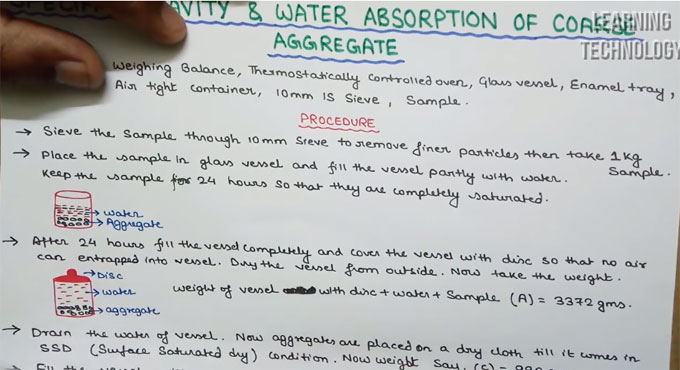# Specific Gravity & Water Absorption of Coarse AggregateThis construction video tutorial is useful for concrete mix design. This video tutorial is based on specific gravity and water absorption of course aggregate.

Different types of apparatus like weighing balance, thermostatically controlled oven, glass vessel, enamel tray, air tight container, 10 mm IS sieve, sample are required for this purpose.

The following methods are used :-

Sieve the sample with the help of 10 mm sieve to eliminate thinner particles and then select 1 kg sample. Set the sample in a glass vessel and load the vessel partially with water.

Retain the sample for 24 hours in order that these are fully soaked.

After completion of 24 hours, load the vessel entirely and cover the vessel by disc to resist entrapping of air into vessel. Dehydrate the vessel from outside. Now measure the weight.

Weight of vessel with disc + water + sample (A) = 3372 gms.

Discharge the water of vessel. Now aggregates are set on a dry cloth unless it comes in SSD (surface saturated dry) condition. Now take the weight as C = 990 gms.

Now load the vessel fully with water. Cover the vessel with disc so that no air can enter into it. Now, again take the weight.

Weight of vessel together with disc and water (B) = 2574 gms.

Now, put the SSD sample in a tray made of enamel and retain it in oven at 1000C for 24 hours. Once 24 hours are completed, let the sample cool in air tight container.

Now, take the weight of the oven dry sample as D = 982 gms.

The following formula is used to determine the specific gravity :-

D/C-(A-B), here, A denotes weight of vessel with disc + water + sample = 3372 gms
B denotes weight of vessel with disc + water = 2754 gms

C denotes weight of SSD sample = 990 gms
D denotes weight of oven dry sample = 982 gms

To determine water absorption, use the following formula :-
C-D/D x 100 = 990 – 982/982 x 100 = 0.81%

To get more detail information, go through the following video tutorial.

Video Source: L & T - Learning Technology